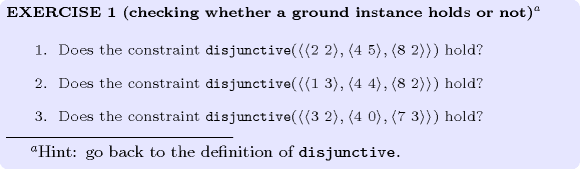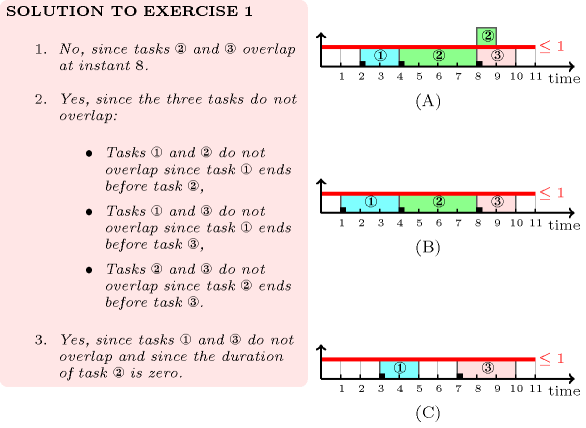## 5.126. disjunctive

Origin
Constraint

$\mathrm{𝚍𝚒𝚜𝚓𝚞𝚗𝚌𝚝𝚒𝚟𝚎}\left(\mathrm{𝚃𝙰𝚂𝙺𝚂}\right)$

Synonym

$\mathrm{𝚘𝚗𝚎}_\mathrm{𝚖𝚊𝚌𝚑𝚒𝚗𝚎}$.

Argument
 $\mathrm{𝚃𝙰𝚂𝙺𝚂}$ $\mathrm{𝚌𝚘𝚕𝚕𝚎𝚌𝚝𝚒𝚘𝚗}\left(\mathrm{𝚘𝚛𝚒𝚐𝚒𝚗}-\mathrm{𝚍𝚟𝚊𝚛},\mathrm{𝚍𝚞𝚛𝚊𝚝𝚒𝚘𝚗}-\mathrm{𝚍𝚟𝚊𝚛}\right)$
Restrictions
 $\mathrm{𝚛𝚎𝚚𝚞𝚒𝚛𝚎𝚍}$$\left(\mathrm{𝚃𝙰𝚂𝙺𝚂},\left[\mathrm{𝚘𝚛𝚒𝚐𝚒𝚗},\mathrm{𝚍𝚞𝚛𝚊𝚝𝚒𝚘𝚗}\right]\right)$ $\mathrm{𝚃𝙰𝚂𝙺𝚂}.\mathrm{𝚍𝚞𝚛𝚊𝚝𝚒𝚘𝚗}\ge 0$
Purpose

All the tasks of the collection $\mathrm{𝚃𝙰𝚂𝙺𝚂}$ that have a duration strictly greater than 0 should not overlap.

Example
$\left(\begin{array}{c}〈\begin{array}{cc}\mathrm{𝚘𝚛𝚒𝚐𝚒𝚗}-1\hfill & \mathrm{𝚍𝚞𝚛𝚊𝚝𝚒𝚘𝚗}-3,\hfill \\ \mathrm{𝚘𝚛𝚒𝚐𝚒𝚗}-2\hfill & \mathrm{𝚍𝚞𝚛𝚊𝚝𝚒𝚘𝚗}-0,\hfill \\ \mathrm{𝚘𝚛𝚒𝚐𝚒𝚗}-7\hfill & \mathrm{𝚍𝚞𝚛𝚊𝚝𝚒𝚘𝚗}-2,\hfill \\ \mathrm{𝚘𝚛𝚒𝚐𝚒𝚗}-4\hfill & \mathrm{𝚍𝚞𝚛𝚊𝚝𝚒𝚘𝚗}-1\hfill \end{array}〉\hfill \end{array}\right)$

Figure 5.126.1 shows the tasks with non-zero duration of the example. Since these tasks do not overlap the $\mathrm{𝚍𝚒𝚜𝚓𝚞𝚗𝚌𝚝𝚒𝚟𝚎}$ constraint holds.

##### Figure 5.126.1. Tasks with non-zero duration of the Example slot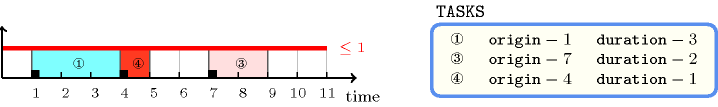All solutions

Figure 5.126.2 gives all solutions to the following non ground instance of the $\mathrm{𝚍𝚒𝚜𝚓𝚞𝚗𝚌𝚝𝚒𝚟𝚎}$ constraint: ${O}_{1}\in \left[2,5\right]$, ${D}_{1}\in \left[2,4\right]$, ${O}_{2}\in \left[2,4\right]$, ${D}_{2}\in \left[1,6\right]$, ${O}_{3}\in \left[3,6\right]$, ${D}_{3}\in \left[4,4\right]$, ${O}_{4}\in \left[2,7\right]$, ${D}_{4}\in \left[1,3\right]$, $\mathrm{𝚍𝚒𝚜𝚓𝚞𝚗𝚌𝚝𝚒𝚟𝚎}$$\left(〈{O}_{1}{D}_{1},{O}_{2}{D}_{2},{O}_{3}{D}_{3},{O}_{4}{D}_{4}〉\right)$.

##### Figure 5.126.2. All solutions corresponding to the non ground example of the $\mathrm{𝚍𝚒𝚜𝚓𝚞𝚗𝚌𝚝𝚒𝚟𝚎}$ constraint of the All solutions slot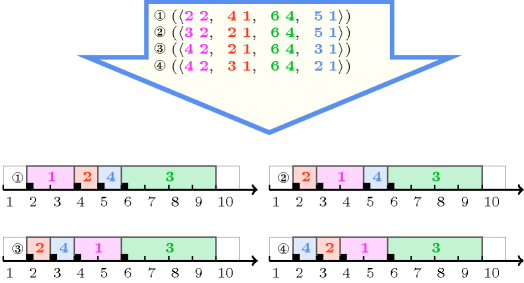Typical
 $|\mathrm{𝚃𝙰𝚂𝙺𝚂}|>2$ $\mathrm{𝚃𝙰𝚂𝙺𝚂}.\mathrm{𝚍𝚞𝚛𝚊𝚝𝚒𝚘𝚗}\ge 1$
Symmetries
• Items of $\mathrm{𝚃𝙰𝚂𝙺𝚂}$ are permutable.

• $\mathrm{𝚃𝙰𝚂𝙺𝚂}.\mathrm{𝚍𝚞𝚛𝚊𝚝𝚒𝚘𝚗}$ can be decreased to any value $\ge 0$.

• One and the same constant can be added to the $\mathrm{𝚘𝚛𝚒𝚐𝚒𝚗}$ attribute of all items of $\mathrm{𝚃𝙰𝚂𝙺𝚂}$.

Arg. properties

Contractible wrt. $\mathrm{𝚃𝙰𝚂𝙺𝚂}$.

Usage

The $\mathrm{𝚍𝚒𝚜𝚓𝚞𝚗𝚌𝚝𝚒𝚟𝚎}$ constraint occurs in many resource scheduling problems in order to model a resource that can not be shared. This means that tasks using this resource can not overlap in time. Quite often $\mathrm{𝚍𝚒𝚜𝚓𝚞𝚗𝚌𝚝𝚒𝚟𝚎}$ constraints are used together with precedence constraints. A precedence constraint between two tasks models the fact that the processing of a task has to be postponed until an other task is completed. Such mix of disjunctive and precedence constraints occurs for instance in job-shop problems.

Remark

Some systems like Ilog CP Optimizer also imposes that zero duration tasks do not overlap non-zero duration tasks.

A soft version of this constraint, under the hypothesis that all durations are fixed, was presented by P. Baptiste et al. in [BaptisteLePapePeridy98]. In this context the goal was to perform as many tasks as possible within their respective due-dates.

When all tasks have the same (fixed) duration the $\mathrm{𝚍𝚒𝚜𝚓𝚞𝚗𝚌𝚝𝚒𝚟𝚎}$ constraint can be reformulated as an $\mathrm{𝚊𝚕𝚕}_\mathrm{𝚖𝚒𝚗}_\mathrm{𝚍𝚒𝚜𝚝}$ constraint for which a filtering algorithm achieving bound-consistency is available [ArtiouchineBaptiste05].

Within the context of linear programming [Hooker07book] provides several relaxations of the $\mathrm{𝚍𝚒𝚜𝚓𝚞𝚗𝚌𝚝𝚒𝚟𝚎}$ constraint.

Some solvers use in a pre-processing phase, while stating precedence and cumulative constraints, an algorithm for automatically extracting large cliques [BronKerbosch73] from a set of tasks that should not pairwise overlap (i.e., two tasks ${t}_{i}$ and ${t}_{j}$ can not overlap either, because ${t}_{i}$ ends before the start of ${t}_{j}$, either because the sum of resource consumption of ${t}_{i}$ and ${t}_{j}$ exceeds the capacity of a cumulative resource that both tasks use) in order to state $\mathrm{𝚍𝚒𝚜𝚓𝚞𝚗𝚌𝚝𝚒𝚟𝚎}$ constraints.

Algorithm

We have four main families of methods for handling the $\mathrm{𝚍𝚒𝚜𝚓𝚞𝚗𝚌𝚝𝚒𝚟𝚎}$ constraint:

• Methods based on the compulsory part [Lahrichi82] of the tasks (also called time-tabling methods). These methods determine the time slots which for sure are occupied by a given task, an propagate back this information to the attributes of each task (i.e., the origin and the duration). Because of their simplicity, these methods have been originally used for handling the $\mathrm{𝚍𝚒𝚜𝚓𝚞𝚗𝚌𝚝𝚒𝚟𝚎}$ constraint. Even if they propagate less than the other methods they can in practice handle a large number of tasks. To our best knowledge no efficient incremental algorithm devoted to this problem was published up to now (i.e., September 2006).

• Methods based on constructive disjunction. The idea is to try out each alternative of a disjunction (e.g., given two tasks ${t}_{1}$ and ${t}_{2}$ that should not overlap, we successively assume that ${t}_{1}$ finishes before ${t}_{2}$, and that ${t}_{2}$ finishes before ${t}_{1}$) and to remove values that were pruned in both alternatives.

• Methods based on edge-finding. Given a set of tasks $𝒯$, edge-finding determines that some task must, can, or cannot execute first or last in $𝒯$. Efficient edge-finding algorithms for handling the $\mathrm{𝚍𝚒𝚜𝚓𝚞𝚗𝚌𝚝𝚒𝚟𝚎}$ constraint were originally described in [CarlierPinson88], [CarlierPinson90] and more recently in [Vilim04], [PeridyRivreau05].

• Methods that, for any task $t$, consider the maximal number of tasks that can end up before the start of task $t$ as well as the maximal number of tasks that can start after the end of task $t$ [Wolf05].

All these methods are usually used for adjusting the minimum and maximum values of the variables of the $\mathrm{𝚍𝚒𝚜𝚓𝚞𝚗𝚌𝚝𝚒𝚟𝚎}$ constraint. However some systems use these methods for pruning the full domain of the variables. Finally, Jackson priority rule [Jackson55] provides a necessary condition [CarlierPinson90] for the $\mathrm{𝚍𝚒𝚜𝚓𝚞𝚗𝚌𝚝𝚒𝚟𝚎}$ constraint. Given a set of tasks $𝒯$, it consists to progressively schedule all tasks of $𝒯$ in the following way:

• It assigns to the first possible time point (i.e., the earliest start of all tasks of $𝒯$) the available task with minimal latest end. In this context, available means a task for which the earliest start is less than or equal to the considered time point.

• It continues by considering the next time point until all the tasks are completely scheduled.

Systems

generalisation: $\mathrm{𝚌𝚞𝚖𝚞𝚕𝚊𝚝𝚒𝚟𝚎}$ ($\mathrm{𝚝𝚊𝚜𝚔}$ $\mathrm{𝚑𝚎𝚒𝚐𝚑𝚝𝚜}$ and $\mathrm{𝚛𝚎𝚜𝚘𝚞𝚛𝚌𝚎}$ $\mathrm{𝚕𝚒𝚖𝚒𝚝}$ are not necessarly all equal to 1), $\mathrm{𝚍𝚒𝚏𝚏𝚗}$ ($\mathrm{𝚝𝚊𝚜𝚔}$ of $\mathrm{𝚑𝚎𝚒𝚐𝚝𝚑}$ 1 replaced by orthotope).

specialisation: $\mathrm{𝚊𝚕𝚕}_\mathrm{𝚖𝚒𝚗}_\mathrm{𝚍𝚒𝚜𝚝}$ ($\mathrm{𝚕𝚒𝚗𝚎}\mathrm{𝚜𝚎𝚐𝚖𝚎𝚗𝚝}$ replaced by $\mathrm{𝚕𝚒𝚗𝚎}\mathrm{𝚜𝚎𝚐𝚖𝚎𝚗𝚝}$, of same length), $\mathrm{𝚊𝚕𝚕𝚍𝚒𝚏𝚏𝚎𝚛𝚎𝚗𝚝}$ ($\mathrm{𝚝𝚊𝚜𝚔}$ replaced by $\mathrm{𝚟𝚊𝚛𝚒𝚊𝚋𝚕𝚎}$).

Keywords
Cond. implications

$•$ $\mathrm{𝚍𝚒𝚜𝚓𝚞𝚗𝚌𝚝𝚒𝚟𝚎}\left(\mathrm{𝚃𝙰𝚂𝙺𝚂}\right)$

with  $\mathrm{𝚖𝚒𝚗𝚟𝚊𝚕}$$\left(\mathrm{𝚃𝙰𝚂𝙺𝚂}.\mathrm{𝚍𝚞𝚛𝚊𝚝𝚒𝚘𝚗}\right)>0$

implies $\mathrm{𝚊𝚕𝚕𝚍𝚒𝚏𝚏𝚎𝚛𝚎𝚗𝚝}$$\left(\mathrm{𝚃𝙰𝚂𝙺𝚂}.\mathrm{𝚘𝚛𝚒𝚐𝚒𝚗}\right)$.

$•$ $\mathrm{𝚍𝚒𝚜𝚓𝚞𝚗𝚌𝚝𝚒𝚟𝚎}\left(\mathrm{𝚃𝙰𝚂𝙺𝚂}\right)$

with  $\mathrm{𝚖𝚒𝚗𝚟𝚊𝚕}$$\left(\mathrm{𝚃𝙰𝚂𝙺𝚂}.\mathrm{𝚍𝚞𝚛𝚊𝚝𝚒𝚘𝚗}\right)>0$

implies $\mathrm{𝚊𝚕𝚕𝚍𝚒𝚏𝚏𝚎𝚛𝚎𝚗𝚝}_\mathrm{𝚌𝚜𝚝}$$\left(\mathrm{𝚅𝙰𝚁𝙸𝙰𝙱𝙻𝙴𝚂}:\mathrm{𝚃𝙰𝚂𝙺𝚂}\right)$.

Arc input(s)

$\mathrm{𝚃𝙰𝚂𝙺𝚂}$

Arc generator
$\mathrm{𝐶𝐿𝐼𝑄𝑈𝐸}$$\left(<\right)↦\mathrm{𝚌𝚘𝚕𝚕𝚎𝚌𝚝𝚒𝚘𝚗}\left(\mathrm{𝚝𝚊𝚜𝚔𝚜}\mathtt{1},\mathrm{𝚝𝚊𝚜𝚔𝚜}\mathtt{2}\right)$

Arc arity
Arc constraint(s)
$\bigvee \left(\begin{array}{c}\mathrm{𝚝𝚊𝚜𝚔𝚜}\mathtt{1}.\mathrm{𝚍𝚞𝚛𝚊𝚝𝚒𝚘𝚗}=0,\hfill \\ \mathrm{𝚝𝚊𝚜𝚔𝚜}\mathtt{2}.\mathrm{𝚍𝚞𝚛𝚊𝚝𝚒𝚘𝚗}=0,\hfill \\ \mathrm{𝚝𝚊𝚜𝚔𝚜}\mathtt{1}.\mathrm{𝚘𝚛𝚒𝚐𝚒𝚗}+\mathrm{𝚝𝚊𝚜𝚔𝚜}\mathtt{1}.\mathrm{𝚍𝚞𝚛𝚊𝚝𝚒𝚘𝚗}\le \mathrm{𝚝𝚊𝚜𝚔𝚜}\mathtt{2}.\mathrm{𝚘𝚛𝚒𝚐𝚒𝚗},\hfill \\ \mathrm{𝚝𝚊𝚜𝚔𝚜}\mathtt{2}.\mathrm{𝚘𝚛𝚒𝚐𝚒𝚗}+\mathrm{𝚝𝚊𝚜𝚔𝚜}\mathtt{2}.\mathrm{𝚍𝚞𝚛𝚊𝚝𝚒𝚘𝚗}\le \mathrm{𝚝𝚊𝚜𝚔𝚜}\mathtt{1}.\mathrm{𝚘𝚛𝚒𝚐𝚒𝚗}\hfill \end{array}\right)$
Graph property(ies)
$\mathrm{𝐍𝐀𝐑𝐂}$$=|\mathrm{𝚃𝙰𝚂𝙺𝚂}|*\left(|\mathrm{𝚃𝙰𝚂𝙺𝚂}|-1\right)/2$

Graph model

We generate a clique with a non-overlapping constraint between each pair of distinct tasks and state that the number of arcs of the final graph should be equal to the number of arcs of the initial graph.

Parts (A) and (B) of Figure 5.126.3 respectively show the initial and final graph associated with the Example slot. The $\mathrm{𝚍𝚒𝚜𝚓𝚞𝚗𝚌𝚝𝚒𝚟𝚎}$ constraint holds since all the arcs of the initial graph belong to the final graph: all the non-overlapping constraints holds.

##### Figure 5.126.3. Initial and final graph of the $\mathrm{𝚍𝚒𝚜𝚓𝚞𝚗𝚌𝚝𝚒𝚟𝚎}$ constraint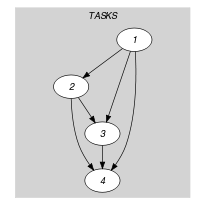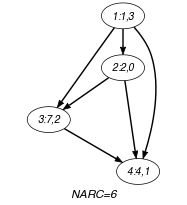(a) (b)
Quiz

$\mathrm{𝚍𝚒𝚜𝚓𝚞𝚗𝚌𝚝𝚒𝚟𝚎}$: checking whether a ground instance holds or not